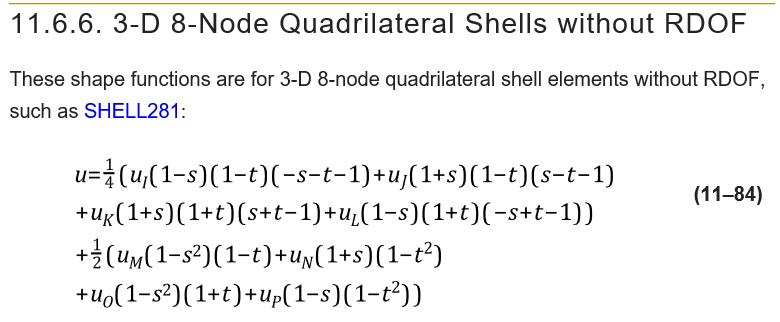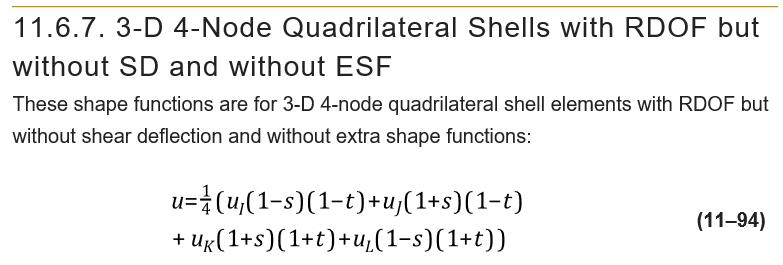## General Mechanical

•JWagoner
Subscriber

• I am attempting to verify the FEA results using a finite difference method in MATLAB

• The model involves a thin plate fully clamped on all sides with a transverse pressure load applied to every node

• In the FEA model, the "sf" command is used on a SOLID186 element type; however, the MATLAB code requires an equation for the uniform pressure to be applied to each finite difference node

• Typically the pressure is approximated as a sinusoidal distribution or a polynomial distribution, does anyone have any insight on which one ANSYS most nearly correlates to in this analysis

Any assistance or resources would be much appreciated.

•Sandeep Medikonda
Ansys Employee

I am not sure, I understand your problem here. Especially without any pictures. Please see this post on the resources available to you.

Regards,
Sandeep

•peteroznewman
Subscriber

JW,

If you have a thin plate, why not use SHELL elements instead of SOLID elements?

If you are trying to match a Finite Difference solver, why not use linear elements like SOLID185 instead of quadratic elements like SOLID186?

An advantage of the linear elements is the shape functions are simpler than the quadratic elements.

You can obtain the shape functions for the face of a solid or shell in the Theory manual.The linear shape function is simpler.Regards,
Peter